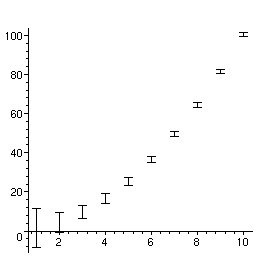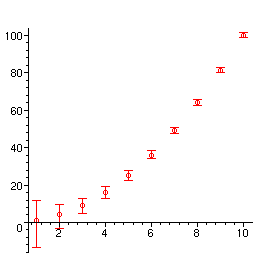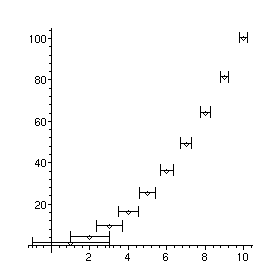Function: errorbar - plot with error bars

Calling Sequences:

errorbar([x,y,er], options);
errorbar([x,y,erplus,erminus], options);

errorbar(L, options);

Parameters:

x,y, er, erplus, erminus - real numbers

L - a set or list of error bar specifications, each in the form [x, y, er] or

[x, y, erplus, erminus]

options - two-dimensional plot options, as well as horizontal and

ticksize= size.

Description:

This creates a two-dimensional plot consisting of one or more error bars.

In the form errorbar([x,y,er]) , the error bar extends from the point [x,y-er] to [x,y+er] . Thus this form is appropriate for a symmetrical data point y ± er .

In the form errorbar([x,y,erplus,erminus]) , the error bar extends from [x,y+erminus] to [x,y+erplus] . Normally, one of erplus and erminus is positive and the other negative, but this is not checked.

If the option horizontal or horizontal=true is included, the error bar is horizontal instead of vertical, i.e. it extends from [x-er,y] to [x+er,y] or [x+erminus,y] to [x+erplus,y] .

Nothing is plotted at [x,y] itself, unless you include the symbol= option, which will plot a symbol at that point. Possible values are BOX , CROSS , CIRCLE , POINT and DIAMOND (but POINT is not very useful).

The ticksize= option specifies the length of the tick marks at the end of the error bars, in terms of the horizontal or vertical distance scale. Set this to 0 to have no tick marks. If this option is omitted, a default length will be used.

As in other plotting commands, the result of errorbar is a PLOT data structure. This can be assigned to a variable, saved in a file, etc.

This function is part of the Maple Advisor Database library, and must be loaded before use by the command readlib(errorbar); .

Examples:

> errorbar([seq([i,i^2,10/i],i=1..10)]);> errorbar([seq([i,i^2,-15/i,10/i],i=1..10)],symbol=circle,colour=red);> errorbar([seq([i,i^2,2/i],i=1..10)],symbol=diamond,horizontal,ticksize=5);